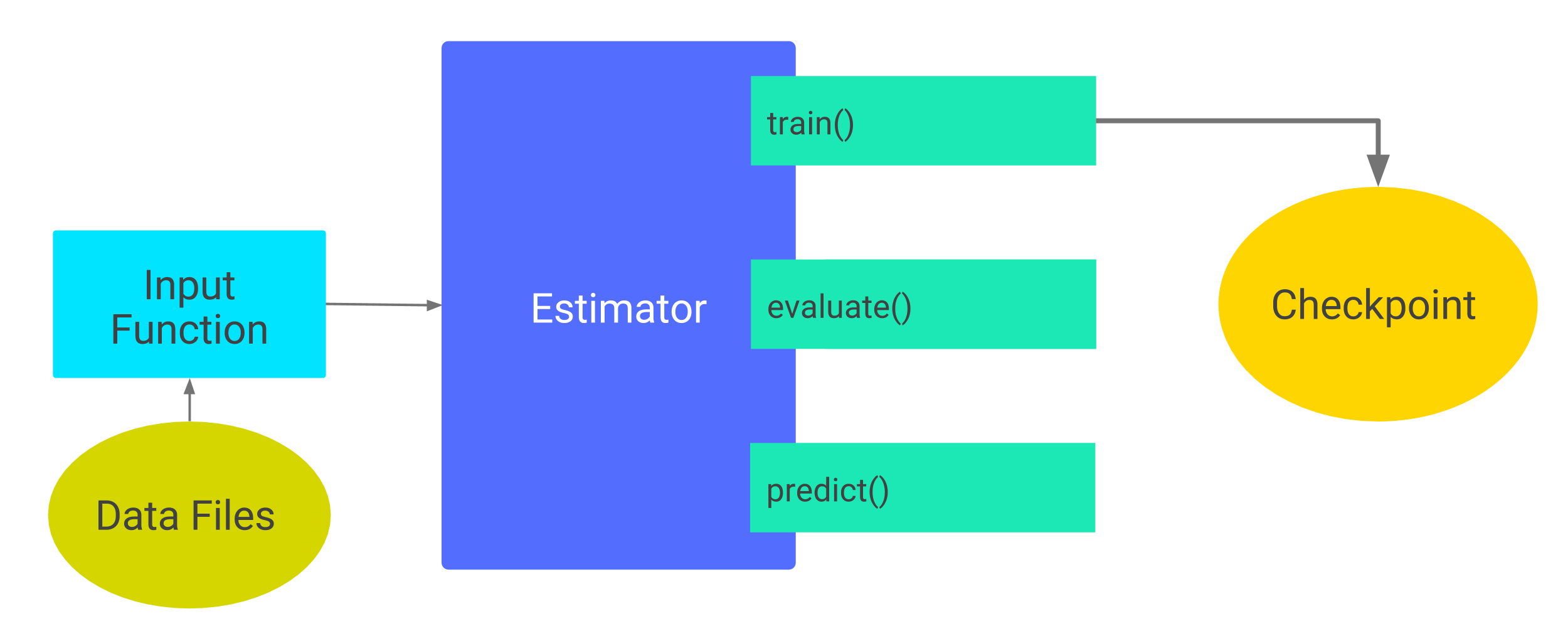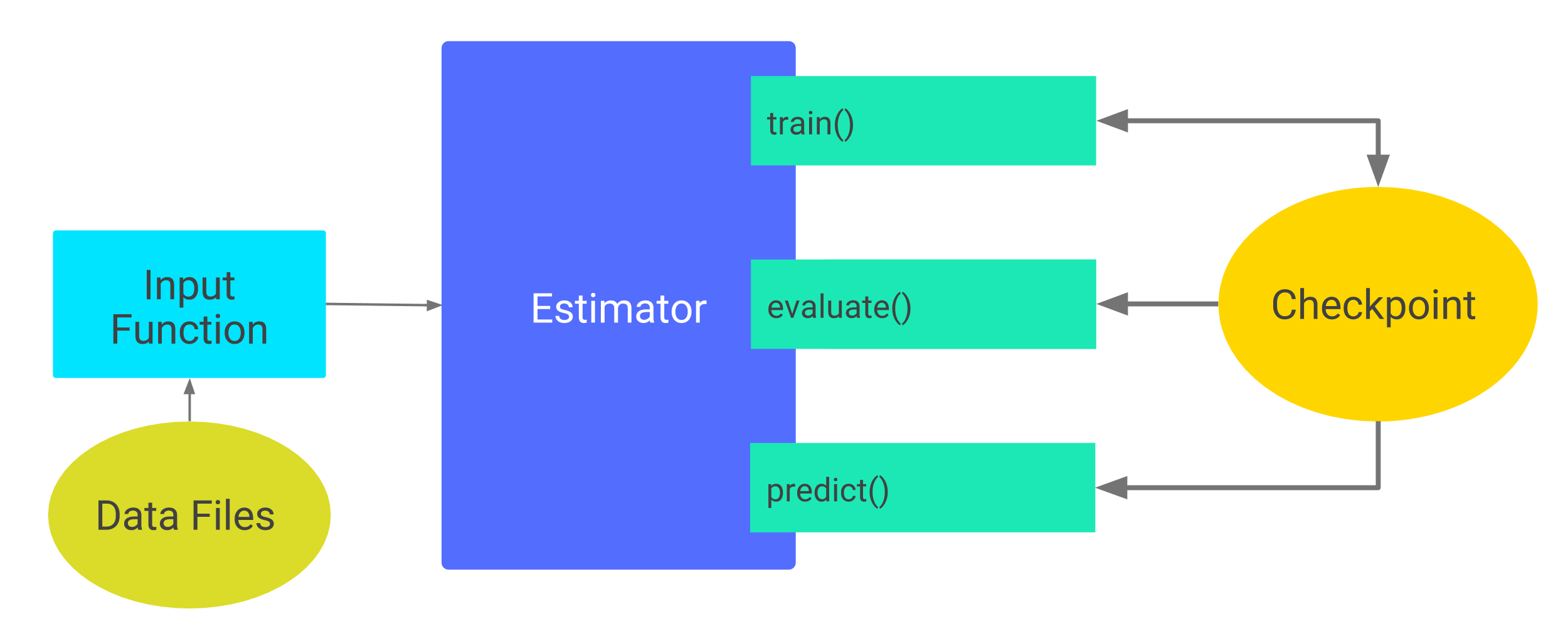TensorFlow 2.0 RC is available

# 检查点

• 检查点：这种格式依赖于创建模型的代码。
• SavedModel：这种格式与创建模型的代码无关。

## 示例代码

git clone https://github.com/tensorflow/models/
cd models/samples/core/get_started


## 保存经过部分训练的模型

Estimator 自动将以下内容写入磁盘：

• 检查点：训练期间所创建的模型版本。
• 事件文件：其中包含 TensorBoard 用于创建可视化图表的信息。

classifier = tf.estimator.DNNClassifier(
feature_columns=my_feature_columns,
hidden_units=[10, 10],
n_classes=3,
model_dir='models/iris')


classifier.train(
input_fn=lambda:train_input_fn(train_x, train_y, batch_size=100),
steps=200)\$ ls -1 models/iris
checkpoint
events.out.tfevents.timestamp.hostname
graph.pbtxt
model.ckpt-1.data-00000-of-00001
model.ckpt-1.index
model.ckpt-1.meta
model.ckpt-200.data-00000-of-00001
model.ckpt-200.index
model.ckpt-200.meta


### 默认检查点目录

classifier = tf.estimator.DNNClassifier(
feature_columns=my_feature_columns,
hidden_units=[10, 10],
n_classes=3)

print(classifier.model_dir)


tempfile.mkdtemp 函数会根据您的操作系统选择安全的临时目录。例如，Mac 操作系统上的典型临时目录可能如下所示：

/var/folders/0s/5q9kfzfj3gx2knj0vj8p68yc00dhcr/T/tmpYm1Rwa


### 检查点频率

• 每 10 分钟（600 秒）写入一个检查点。
• train 方法开始（第一次迭代）和完成（最后一次迭代）时写入一个检查点。
• 只在目录中保留 5 个最近写入的检查点。

1. 创建一个 RunConfig 对象来定义所需的时间安排。
2. 在实例化 Estimator 时，将该 RunConfig 对象传递给 Estimator 的 config 参数。

my_checkpointing_config = tf.estimator.RunConfig(
save_checkpoints_secs = 20*60,  # Save checkpoints every 20 minutes.
keep_checkpoint_max = 10,       # Retain the 10 most recent checkpoints.
)

classifier = tf.estimator.DNNClassifier(
feature_columns=my_feature_columns,
hidden_units=[10, 10],
n_classes=3,
model_dir='models/iris',
config=my_checkpointing_config)


## 恢复模型

1. Estimator 通过运行 model_fn() 构建模型。（要详细了解 model_fn()，请参阅创建自定义 Estimator。）
2. Estimator 根据最近写入的检查点中存储的数据来初始化新模型的权重。### 避免不当恢复

classifier = tf.estimator.DNNClassifier(
feature_columns=feature_columns,
hidden_units=[10, 10],
n_classes=3,
model_dir='models/iris')

classifier.train(
input_fn=lambda:train_input_fn(train_x, train_y, batch_size=100),
steps=200)


classifier2 = tf.estimator.DNNClassifier(
feature_columns=my_feature_columns,
hidden_units=[20, 20],  # Change the number of neurons in the model.
n_classes=3,
model_dir='models/iris')

classifier.train(
input_fn=lambda:train_input_fn(train_x, train_y, batch_size=100),
steps=200)


...
InvalidArgumentError (see above for traceback): tensor_name =